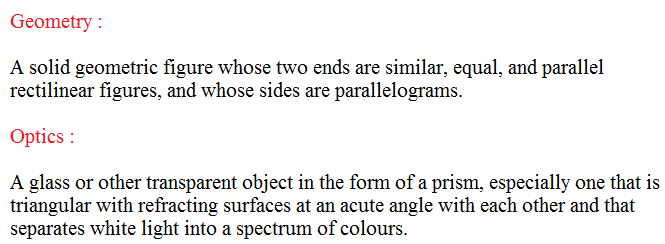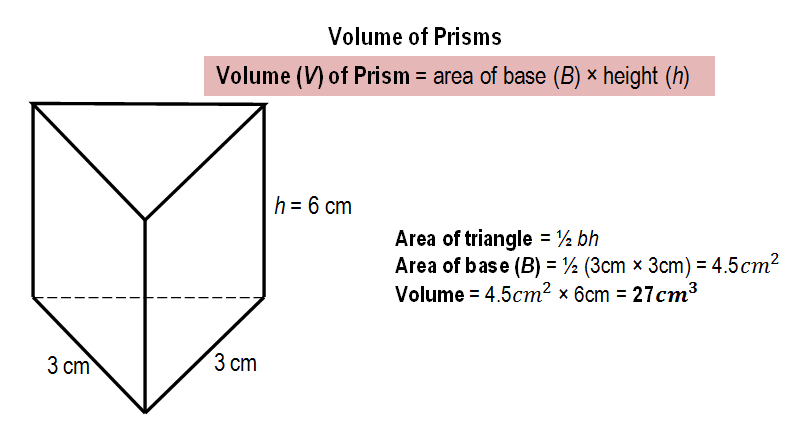# Java Program To Calculate Volume Of Prism | 3 Simple ways

Java program to calculate the volume of a prism. we have been written the java code in three to four different formats. All of the formats are meant to be same just for a learning purpose. If you have any doubt related to the subject that we are shared over here do let us know by commenting or contacting us. We are glad to help you out #java program # #Volume of Prism #.

# Online compiler and execution tool # are added for each type of the program, under each example o code. check it out.

1. Example # 1 – A simple program universally applicable.
2. Example # 2 – Using command line argument # may not universally applicable( subject purpose ).
3. Example # 3 – Using a static method – # Universally applicable.

Before we get into the topic, we just decide to share a couple of lines about a volume of a prism. Here goes!

What is a prism?

Here is the actual and complete exact definition for you :Prism Definition

What is the formula in order to calculate the volume of a prism?

Since in math, there may be several ways to find the volume of a prism, the easiest formula as follows :

The volume of a prism = Area of base X HeightThe volume of prism # Formula

Note: Before calculating the volume of prism, you must calculate the Area of base

Here is the example program # 1 with online compiler and execution tool. Make sure to check out the rest of the examples in different formats to know how the code actually works. If you were at starting level to learn the java then do subscribe to our blog. Our dedicated team will share the best of the information related to coding from a basic level to core level.

Example method # 1: Check out the execution tool as well # volume of prism java code #

Output :

# Here is the online execution & Compiler tool for the above example program -1 #

You may get an idea. How the above code works in order to calculate the volume of a prism. Below is the complete explanation of that java code. Check only, if you were new to programming. If you know the basics of java programming or coding then skip this step and follow out to the next example that we are written as # Example method # 2.

Here is the step by step explanation :

– Scan the input variables and provide the necessary files from inbuilt JDK package. Used in the new scanner method or syntax.

– The class is a blueprint, where objects are created within a class.

– It’s a method, where your string input variables converted to arguments like as double, int, float, whatever you were written in the code.

– The scanner is a method that retrieves the data from the java.util.scanner and uses to read the input variables from your device(system.in).

– Returns the output value on the screen, whatever you have been written in the brackets.

– Double is a method, which can take the variables into decimal points either normal integers. Where ‘ base ‘ names to that memory, where s is a scanner function to let you know that it is an integer. Nextdouble(); represents to store the given value and cursor will move on to the second line until the program completes its execution.

– The formula to find out the volume of a prism in java.

– The output value will be displayed over here.

So, you got an idea how the above java program to calculate the volume of a prism. Did you check out the online compiler tool? Anyways, here is another example method to calculate the volume of a prism in java. Consider the following Java code as an example method # 2.

The little difference, when compares with the above code is nothing but just a small change in syntax. Here a new method called ‘ parse ‘. Maybe the below program cannot execute on your cmd file. Check out the program.

Example program #2 :

Here is the online execution tool for the above program in order to calculate the volume of prism :

The above program may not work on your command line. Since the example program #1 is universally applicable to all and can execute anywhere on JDK. Below is the complete explanation of the above code, parse :

You may get an idea what is parsing and how the parse method works in the above java program to calculate the volume of a prism.

Here is another example program. Check it out, the following program can also universally applicable.

Example Method – # 3

How simple it was right! If you have any doubts related to the above examples and how the java program works. Do comment, we are glad to help you out related to this query.

# Java program to find the volume of prism ends #

x

## Plus Star Pattern Java Program | Patterns

Java program to print plus star pattern program – We have written the below print/draw ...